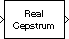# Real Cepstrum

Compute real cepstrum of input

## Library

Transforms

`dspxfrm3`

•## Description

The Real Cepstrum block computes the real cepstrum of each column in the real-valued M-by-N input matrix, `u`. The block treats each column of the input as an independent channel containing M consecutive samples. The block always processes unoriented vector inputs as a single channel, and returns the result as a length-M column vector. The block does not accept complex-valued inputs.

The output is a real Mo-by-N matrix, where you specify Mo in the FFT length parameter. Each output column contains the length-Mo real cepstrum of the corresponding input column.

`y = real(ifft(log(abs(fft(u,Mo)))))`

or, more compactly,

```y = rceps(u,Mo) ```

When you select the Inherit FFT length from input port dimensions check box, the output frame size matches the input frame size (Mo=M).

The output port rate is the same as the input port rate.

## Parameters

Inherit FFT length from input port dimensions

When you select this check box, the output frame size matches the input frame size.

FFT length

The number of frequency points at which to compute the FFT, which is also the output frame size, Mo. This parameter is visible only when you clear the Inherit FFT length from input port dimensions check box.

## Supported Data Types

• Double-precision floating point

• Single-precision floating point

 Complex Cepstrum DSP System Toolbox DCT DSP System Toolbox FFT DSP System Toolbox `rceps` Signal Processing Toolbox

## Extended Capabilities

Introduced before R2006a

## SupportGet trial now